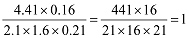# Sample Aptitude Questions of DE-Shaw

1. The HCF and LCM of two numbers are 12 and 924 respectively. Then the number of such pairs is
1. 0
2. 1
3. 2
4. 3
Let the numbers be 12x and 12y where x and y are prime to each other.
∴ LCM = 12xy
∴ 12xy = 924
=> xy = 77
∴ Possible pairs = (1,77) and (7,11)
1. What is the least number which, when divided by 5, 6, 7, 8 gives the remainder 3 but is divisible by 9?
1. 1463
2. 1573
3. 1683
4. 1793
LCM of 5, 6, 7, 8 = 35 × 24 = 840
∴ Required number = 840 k + 3 which is exactly divisible by 9.
For k = 2, it is divisible by 9.
∴ Required number = 840k + 3
= 840 × 2 + 3 = 1683
2. Three numbers are in the ratio 3:4: 5. The sum of the largest and the smallest equals the sum of the second and 52. The smallest number is
1. 20
2. 27
3. 39
4. 52
Let the numbers be 3x, 4x and 5x.
∴ 5x + 3x = 4x + 52
=> 4x = 52 => x =13
∴ The smallest number = 3x = 3 × 13 = 39
3. If the radius of a circle is in creased by 50%, its area is increased by
1. 125%
2. 100%
3. 75%
4. 50%
Percentage increase in area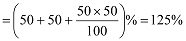4. A and B working separately can do a piece of work in 9 and 12 days respectively. If they work for a day alternately with A beginning, the work would be completed in
1. 10 2/3 days
2. 10 1/2 days
3. 10 1/4 days
4. 10 1/3 days
Part of work done by A and B in first two days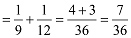Part of work done in first 10 days = 35/36
Remaining work = 1 – 35/36 = 1/36
Now it is the turn of A.
∴ Time taken by A = 1/36 × 9 = ¼
∴ Total time = 10 + ¼ = 10 ¼ days
5. In a family, the average age of a father and a mother is 35 years. The average age of the father, mother and their only son is 27 years. What is the age of the son?
1. 12 years
2. 11 years
3. 10.5 years
4. 10 years
Father + mother = 2 × 35 = 70 years
Father + mother + son = 27 × 3 = 81 years
Son’s age = 81 – 70 = 11 years
6. If 5 men or 7 women can earn Rs. 5,250 per day, how much would 7 men and 13 women earn per day?
1. Rs. 11,600
2. Rs. 11.700
3. Rs. 16,100
4. Rs. 17,100
5 men = 7 women
7 men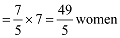Mark Price = 140
7 men + 13 women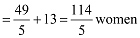Now,
∵ 7 women = Rs. 5250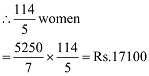7. If A and B together can complete a piece of work in 15 days and B alone in 20 days, in how many days can A alone complete the work?
1. 60
2. 45
3. 40
4. 30
(A + B)’s 1 day’s work =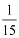B’s 1 day’s work =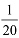A’s 1 day’s work =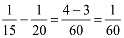A alone will do the work in 60 days.
8. By walking at 3/4 of his usual speed, a man reaches his office 20 minutes later than his usual time. The usual time taken by him to reach his office is
1. 75 minutes
2. 60 minutes
3. 40 minutes
4. 30 minutes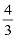of usual time = Usual time + 20 minutes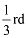of usual time